# How to Create State and County Maps Easily in R

• Last Updated : 24 Oct, 2021

In this article, we will discuss how to create state and county maps in R Programming Language and how to plot such maps with varying color intensities and different use cases.

Maps of Counties and States in a 2D version are simpler and easier to visualize rather than using other conventional visualization methods. If we want to get insights such as which state has the highest population count or in which state the annual budget was the least, for such problem statements maps are the easiest visualization method.

Here we’ll use tidyverse package for utilizing map datasets and ggplot package to plot maps.

## Installation

To install and load tidyverse type the below command in the terminal.

Install the Package – install.packages(“tidyverse”)

## Stepwise Implementation

### Step 1: Load in-built map data

Using map_data( ) function we can load the map datasets that are in-built in tidyverse package.

Syntax: map_data(map, region = “.”, exact = FALSE, …)

Parameters:

• map – name of map provided by the maps package
• region – name of subregions to include
• exact – region as regular expression (FALSE) or as a fixed string (TRUE)

### Example :

In this example, we will load data from state, county USArrests, USA, world, and world2 datasets.

## R

 `# load the package``library``(``"tidyverse"``)`` ` `# Loading map data``states < - ``map_data``(``"state"``)``counties < - ``map_data``(``"county"``)``arrests < - USArrests``usa < -``map_data``(``"usa"``)``world < -``map_data``(``"world"``)``world2 < -``map_data``(``"world2"``)`

### Step 2:  Plot  map data

Here we’ll write a user-defined function in R to plot a map using the above-loaded datasets. We take the dataset, x, y, and column used to fill the map as arguments of the function. Using ggplot( ) function the map is plotted. guides( ) function is used to avoid legends on the map.

Syntax: ggplot(data = NULL, mapping = aes(), …)

Parameters:

• data – dataset
• mapping – aesthetic mappings to use for plot

### Example :

Here, we are writing a function to plot maps using in-built map data

## R

 `# Function to plot maps using in-built map data``map <- ``function``(x,y,dataset,fill_column){``  ``p <- ``ggplot``(data = dataset,``              ``mapping = ``aes``(x = x, y = y, group = group, fill = fill_column))``  ``p + ``geom_polygon``() + ``guides``(fill = ``FALSE``)``}`

### Example: Plotting USA by region on the map

Using the map( ) function created previously, let’s plot a map of the USA. Using the usa dataset and the user-defined function created above, let’s visualize via a plot. We also pass in the longitude , latitude, usa dataset and region column in usa as arguments to the map( ) function.

## R

 `library``(tidyverse)`` ` `# Loading map data``states <- ``map_data``(``"state"``)``counties <- ``map_data``(``"county"``)``arrests <- USArrests``usa <-``map_data``(``"usa"``)``world <-``map_data``(``"world"``)  ``world2<-``map_data``(``"world2"``)`` ` `# Function to plot maps using in-built map data``map <- ``function``(x,y,dataset,fill_column){``  ``p <- ``ggplot``(data = dataset,``              ``mapping = ``aes``(x = x, y = y, group = group, fill = fill_column))``  ``p + ``geom_polygon``() + ``guides``(fill = ``FALSE``)``}`` ` `# entire USA on map``map``(usa\$long,usa\$lat,usa,usa\$region)`

Output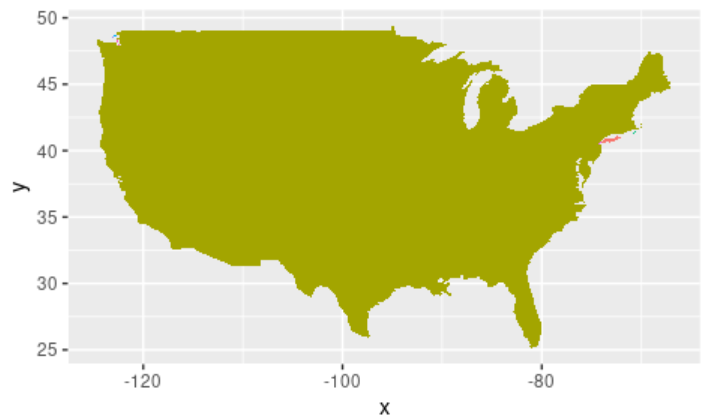### Example: Plotting US States by region on map

Here we’ll fill the USA map state wise which can be visualized with different colors. In the states dataset, using the column “region” we can visualize all of them inside the US map. We also pass in the longitude, latitude, states dataset, and region column in states as arguments to the map( ) function.

## R

 `library``(tidyverse)`` ` `# Loading map data``states <- ``map_data``(``"state"``)``counties <- ``map_data``(``"county"``)``arrests <- USArrests``usa <-``map_data``(``"usa"``)``world <-``map_data``(``"world"``)  ``world2<-``map_data``(``"world2"``)`` ` `# Function to plot maps using in-built map data``map <- ``function``(x,y,dataset,fill_column){``  ``p <- ``ggplot``(data = dataset,``              ``mapping = ``aes``(x = x, y = y, group = group, fill = fill_column))``  ``p + ``geom_polygon``() + ``guides``(fill = ``FALSE``)``}`` ` `# plotting USA states ``map``(states\$long,states\$lat,states,states\$region)`

Output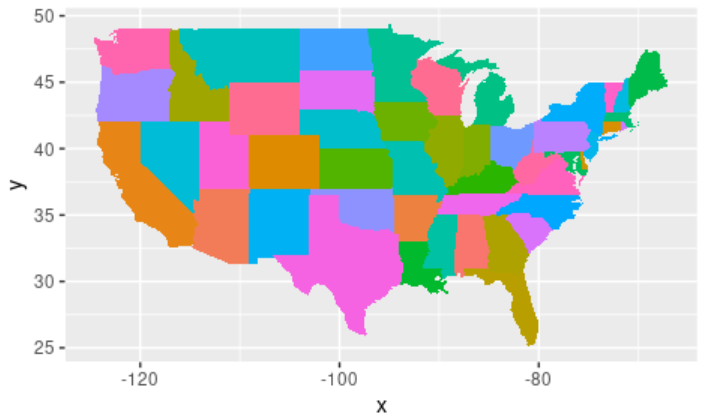### Example: Plotting US counties by subregion with latitude and longitude

Let’s see how to add coordinates to the above map using coord_map( ) function.  This is added to give a globe effect to the map. We can further visualize each subregion inside the USA in each state.

Syntax: coord_map(projection = “mercator”, orientation = NULL,xlim = NULL,ylim = NULL …)

Parameters:

• projection – projection to use
• orientation – projection orientation
• xlim – x limits (in degrees of longitude/latitude)
• ylim – y limits (in degrees of longitude/latitude)

## R

 `library``(tidyverse)`` ` `# Loading map data``states <- ``map_data``(``"state"``)``counties <- ``map_data``(``"county"``)``arrests <- USArrests``usa <-``map_data``(``"usa"``)``world <-``map_data``(``"world"``)  ``world2<-``map_data``(``"world2"``)`` ` `# Function to plot maps using in-built map data``map <- ``function``(x,y,dataset,fill_column){``  ``p <- ``ggplot``(data = dataset,``              ``mapping = ``aes``(x = x, y = y, group = group, fill = fill_column))``  ``p + ``geom_polygon``() + ``guides``(fill = ``FALSE``)``}`` ` `# US counties by subregion with latitude and longitude``map``(counties\$long,counties\$lat,counties,counties\$subregion) +``coord_map``(``"albers"``,  lat0 = 45.5, lat1 = 29.5)`

Output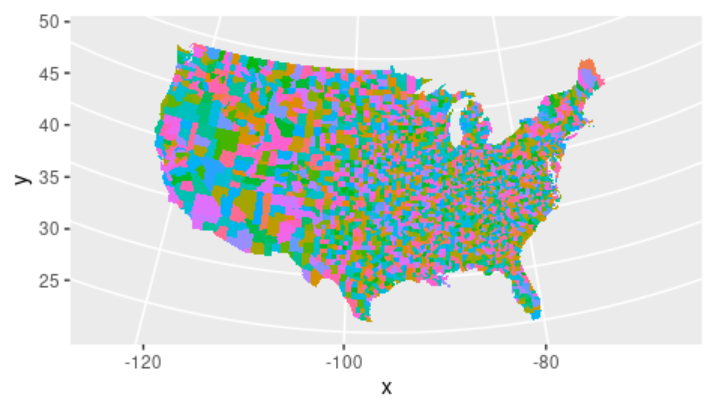### Example: Plotting World map

Here let’s see how to plot the world map using the map( ) function and world dataset. Using the world dataset and the user-defined function defined above, pass in the longitude, latitude, world dataset, and region column in the world as arguments to the map( ) function.

## R

 `library``(tidyverse)`` ` `# Loading map data``states <- ``map_data``(``"state"``)``counties <- ``map_data``(``"county"``)``arrests <- USArrests``usa <-``map_data``(``"usa"``)``world <-``map_data``(``"world"``)  ``world2<-``map_data``(``"world2"``)`` ` `# Function to plot maps using in-built map data``map <- ``function``(x,y,dataset,fill_column){``  ``p <- ``ggplot``(data = dataset,``              ``mapping = ``aes``(x = x, y = y, group = group, fill = fill_column))``  ``p + ``geom_polygon``() + ``guides``(fill = ``FALSE``)``}`` ` `# World Map using world dataset``map``(world\$long,world\$lat,world,world\$region)`

Output: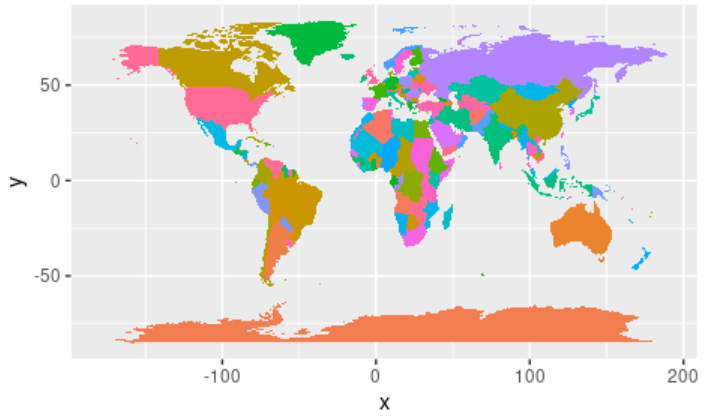### Example: World2 dataset, with a shift in latitude

This is another world map using world2 dataset, with a shift in latitude. Using the map( ) function and world2 dataset, pass in the longitude, latitude, world2 dataset, and region column in world2 as arguments to the map( ) function.

## R

 `library``(tidyverse)`` ` `# Loading map data``states <- ``map_data``(``"state"``)``counties <- ``map_data``(``"county"``)``arrests <- USArrests``usa <-``map_data``(``"usa"``)``world <-``map_data``(``"world"``)  ``world2<-``map_data``(``"world2"``)`` ` `# Function to plot maps using in-built map data``map <- ``function``(x,y,dataset,fill_column){``  ``p <- ``ggplot``(data = dataset,``              ``mapping = ``aes``(x = x, y = y, group = group, fill = fill_column))``  ``p + ``geom_polygon``() + ``guides``(fill = ``FALSE``)``}`` ` `# World Map using world2 dataset``map``(world2\$long,world2\$lat,world2,world2\$region)`

Output: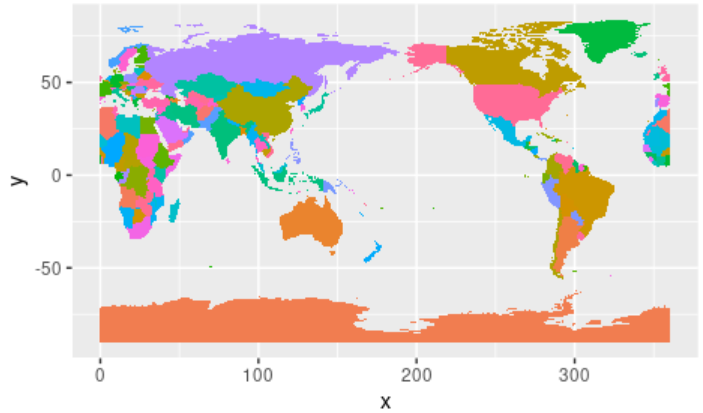My Personal Notes arrow_drop_up